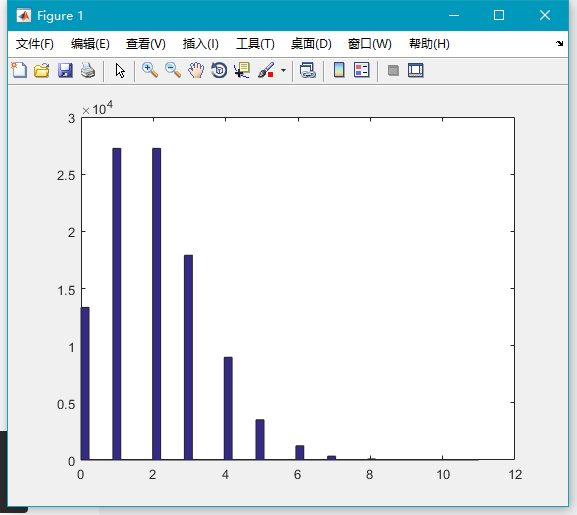# MATLAB 生成随机数 方法总汇 （各分布配图参考）

a. 基本随机数

1．rand()

2．randn()

b. 连续型分布随机数

3．unifrnd()

4．normrnd()

5．chi2rnd()

6．frnd()

7．trnd()

8．betarnd()

10．gamrnd()

11．lognrnd()

12．raylrnd()

c. 离散型分布随机数

14．unidrnd()

15．binornd()

16．geornd()

17．poissrnd()

## a.基本随机数

Matlab 中有两个最基本生成随机数的函数。

### 1．rand()

``rand([M,N,P ...])``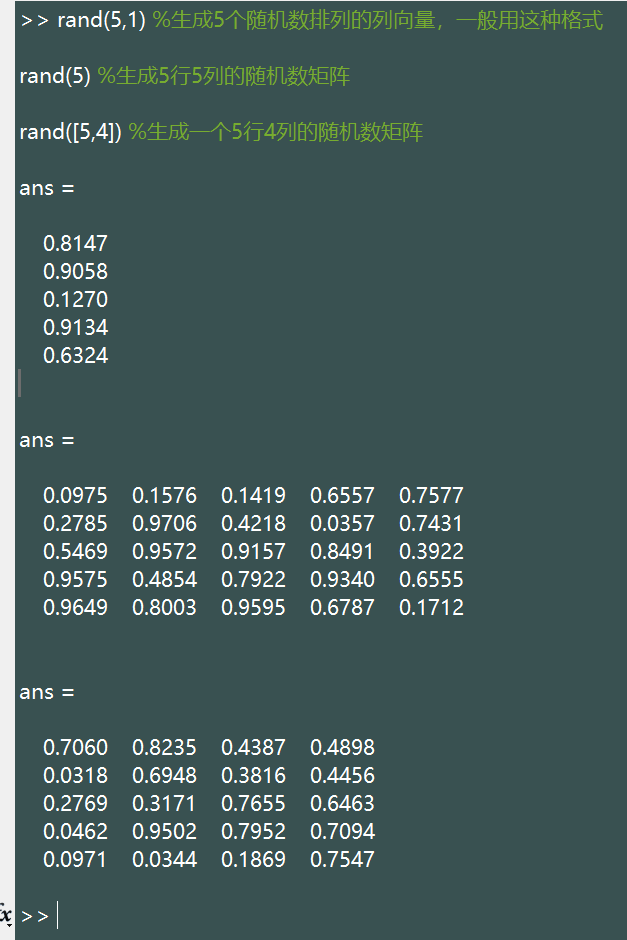``````x=rand(100000,1);

hist(x,30);``````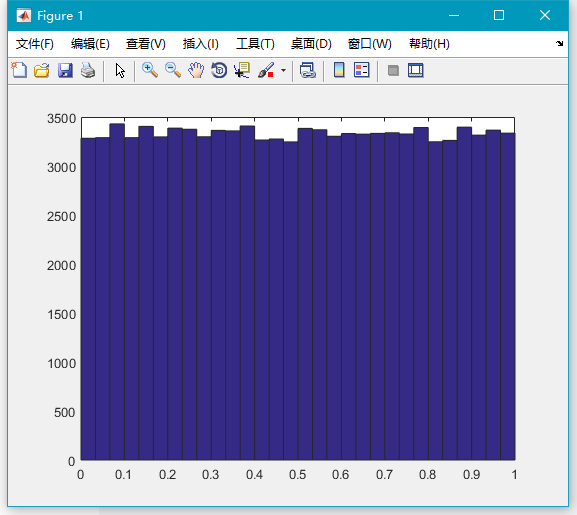### 2．randn()

``randn([M,N,P ...])``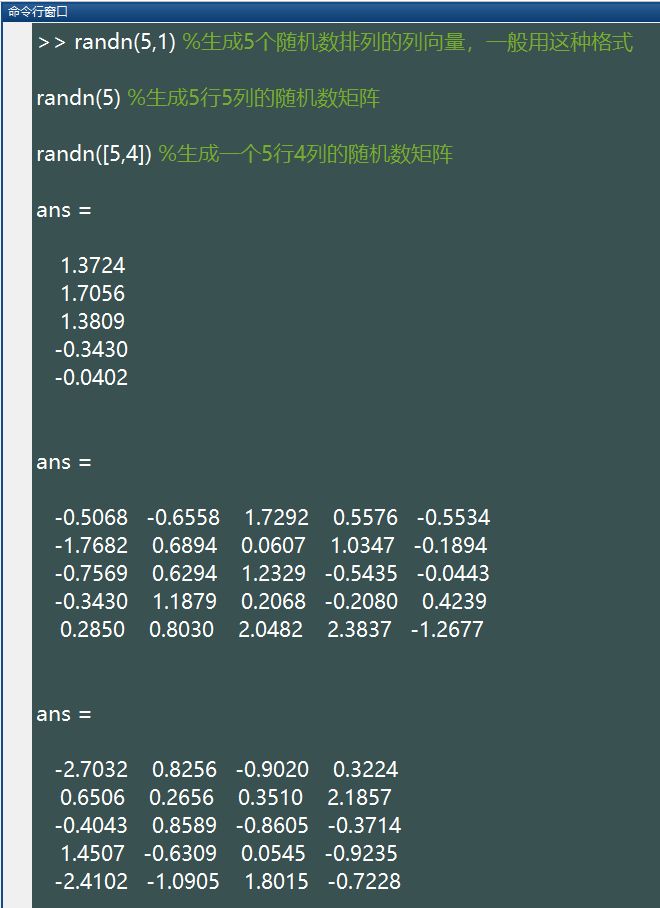``````x=randn(100000,1);

hist(x,50);``````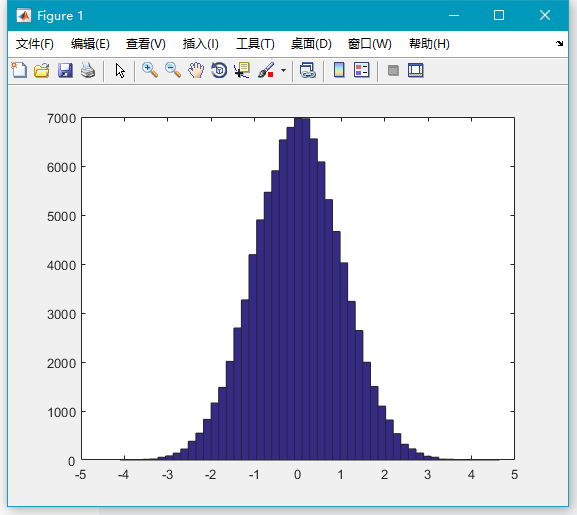## b. 连续型分布随机数

### 3．unifrnd()

``unifrnd(a,b,[M,N,P,...])``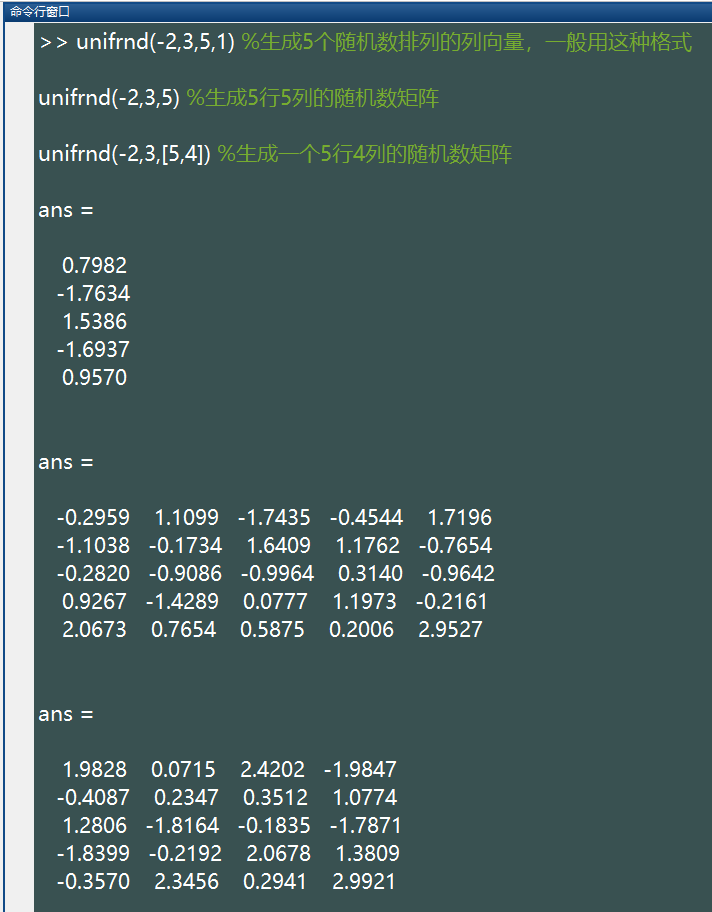%注：上述语句生成的随机数都在 (-2,3) 区间内.

``````x=unifrnd(-2,3,100000,1);

hist(x,50);``````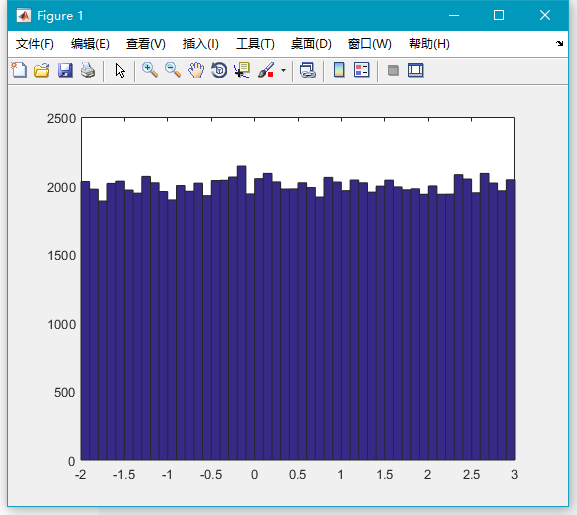### 4．normrnd()

``normrnd(mu,sigma,[M,N,P,...])``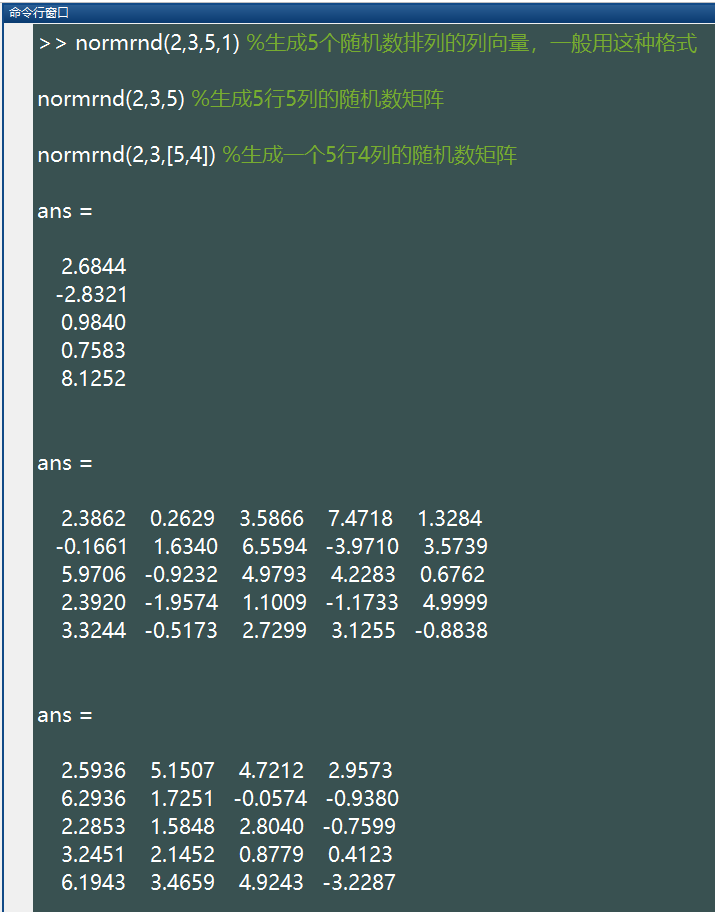%注：上述语句生成的随机数所服从的正态分布都是均值为 2，标准差为 3.

``````x=normrnd(2,3,100000,1);

hist(x,50);``````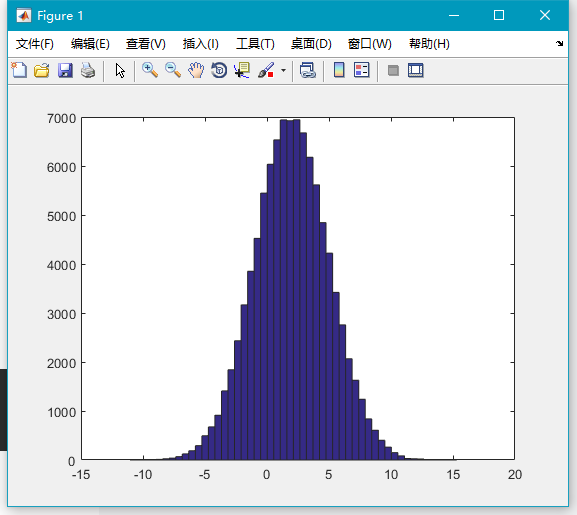### 5．chi2rnd()

``chi2rnd(v,[M,N,P,...])``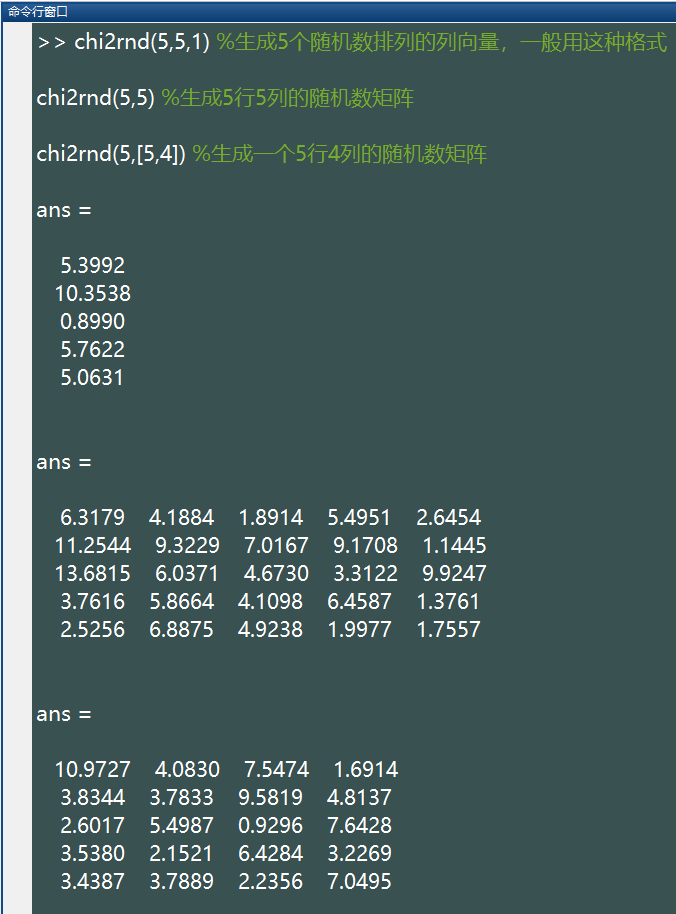%注：上述语句生成的随机数所服从的卡方分布的自由度都是5

``````x=chi2rnd(5,100000,1);

hist(x,50);``````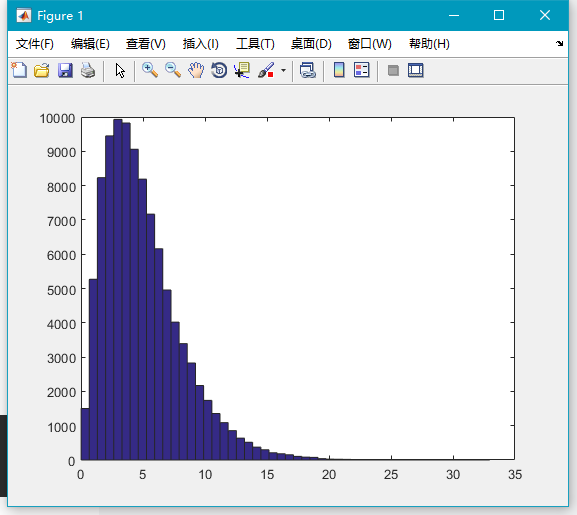### 6．frnd()

``frnd(v1,v2,[M,N,P,...])``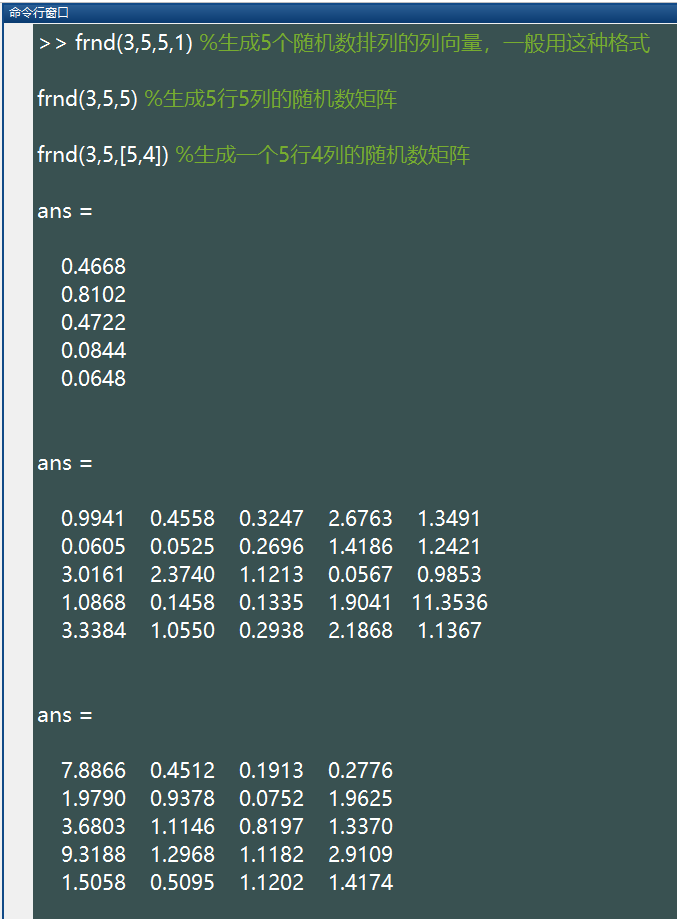%注：上述语句生成的随机数所服从的参数为 (v1=3,v2=5) 的 F 分布

``````x=frnd(3,5,100000,1);

hist(x,50);``````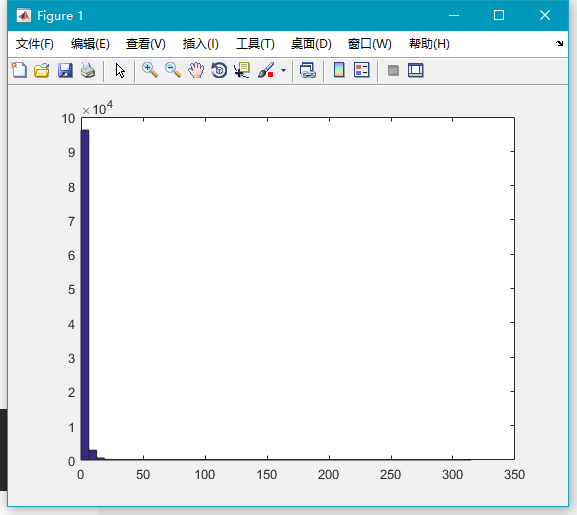### 7．trnd()

``trnd(v,[M,N,P,...])``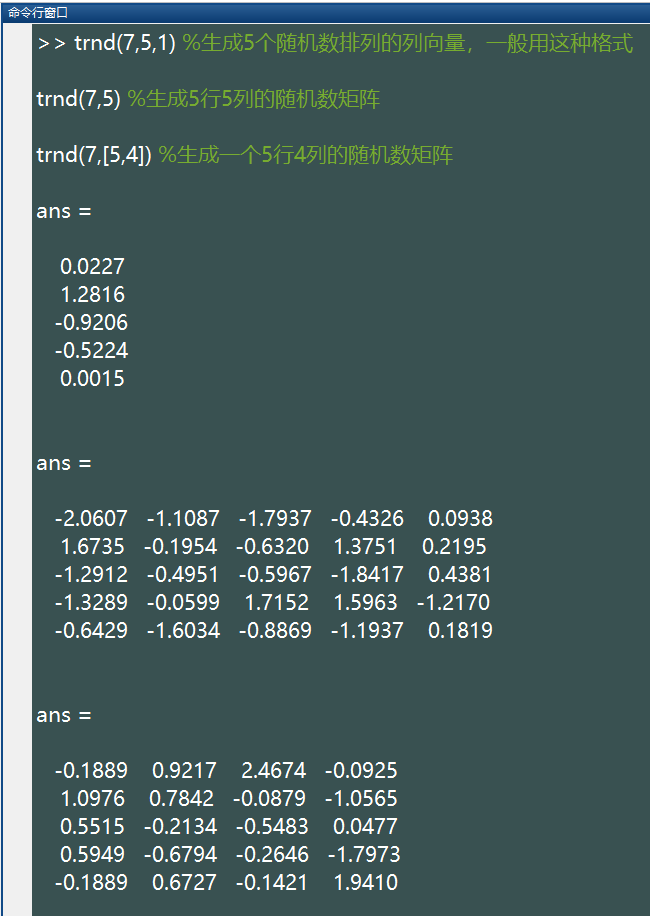%注：上述语句生成的随机数所服从的参数为 (v=7) 的 t 分布

``````x=trnd(7,100000,1);

hist(x,50);``````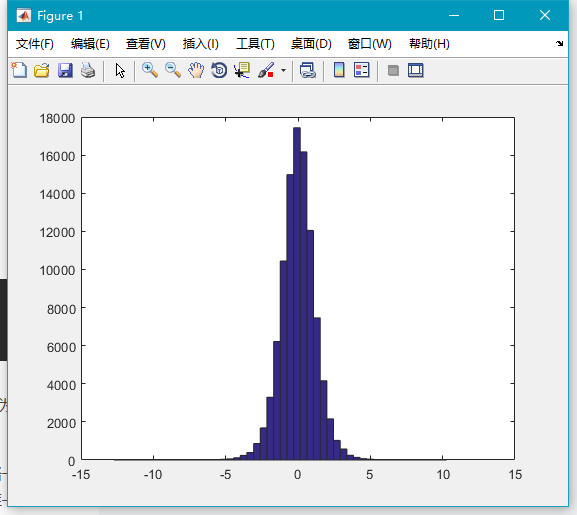### 8．betarnd()

``betarnd(A,B,[M,N,P,...])``

``````x=betarnd(3,5,100000,1);

hist(x,50);``````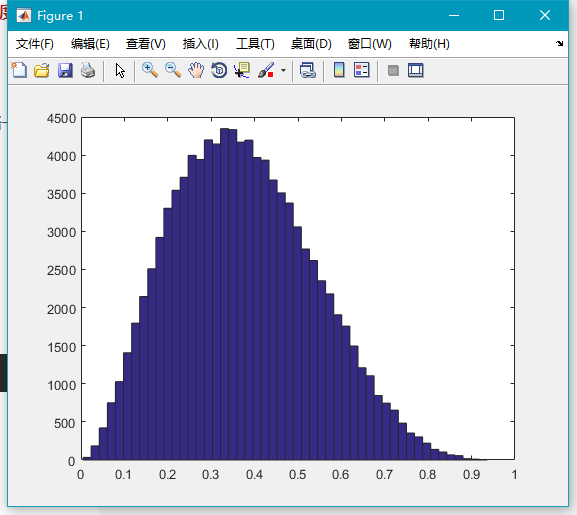9exprnd()

``exprnd(mu,[M,N,P,...])``

``````x=exprnd(0.5,100000,1);

hist(x,50);``````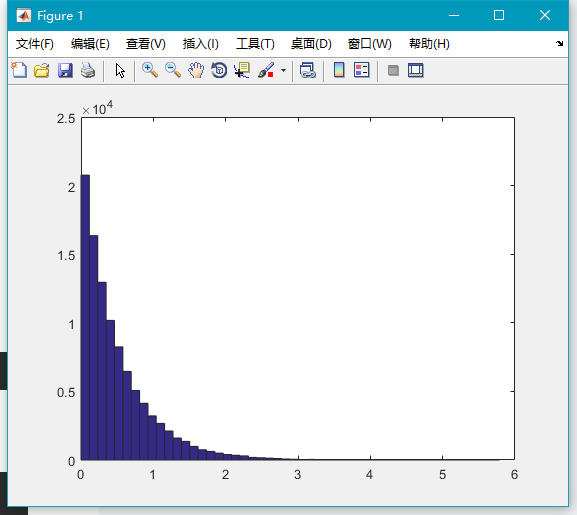### 10．gamrnd()

``gamrnd(A,B,[M,N,P,...])``

``````x=gamrnd(3,5,100000,1);

hist(x,50);``````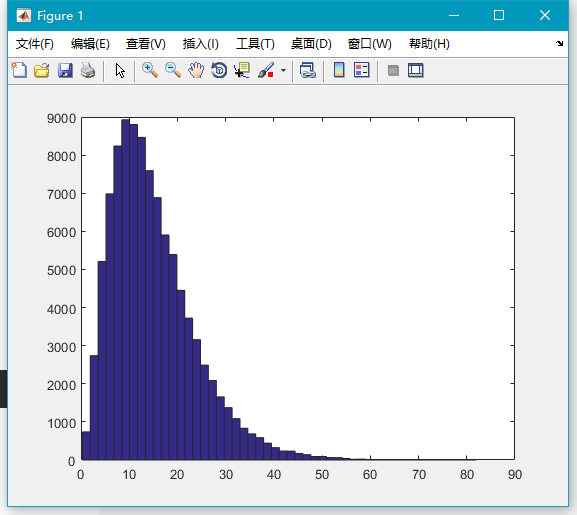### 11．lognrnd()

``lognrnd(mu,sigma,[M,N,P,...])``

``````x=lognrnd(-1,1/1.2,100000,1);

hist(x,50);``````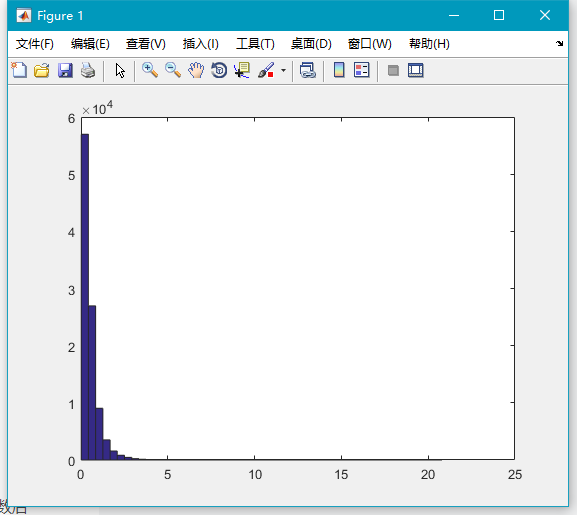### 12．raylrnd()

``raylrnd(B,[M,N,P,...])``

``````x=raylrnd(2,100000,1);

hist(x,50);``````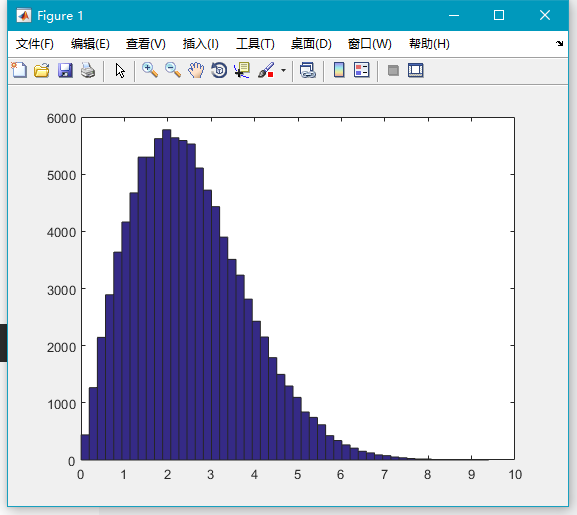13wblrnd()

``wblrnd(A,B,[M,N,P,...])``

``````x=wblrnd(3,2,100000,1);

hist(x,50);``````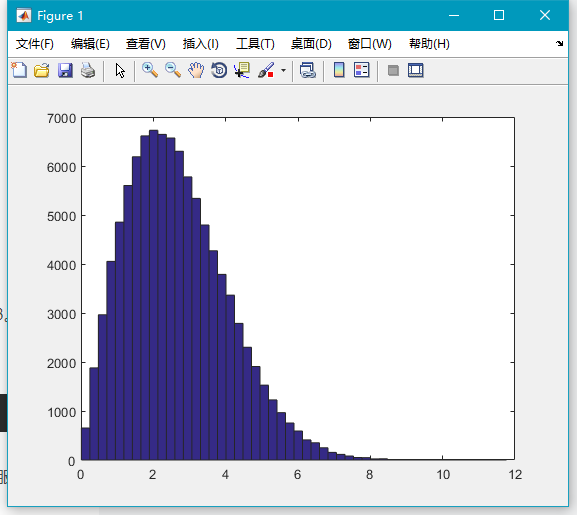## c.离散型分布随机数

### 14．unidrnd()

``unidrnd(n,[M,N,P,...])``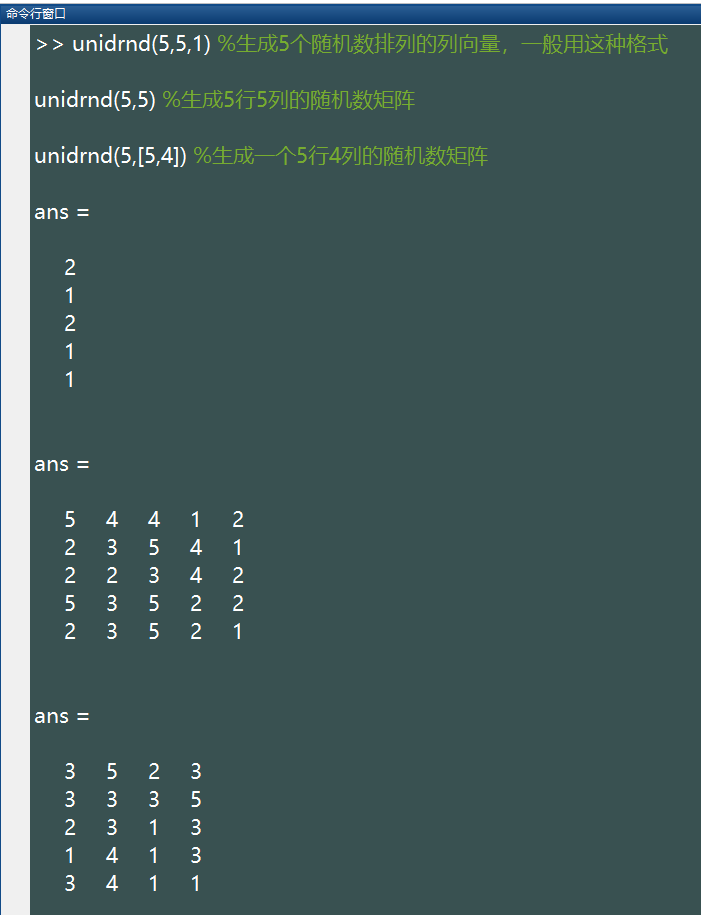%注：上述语句生成的随机数所服从的参数为 (10,0.3) 的二项分布

``````x=unidrnd(9,100000,1);

hist(x,9);``````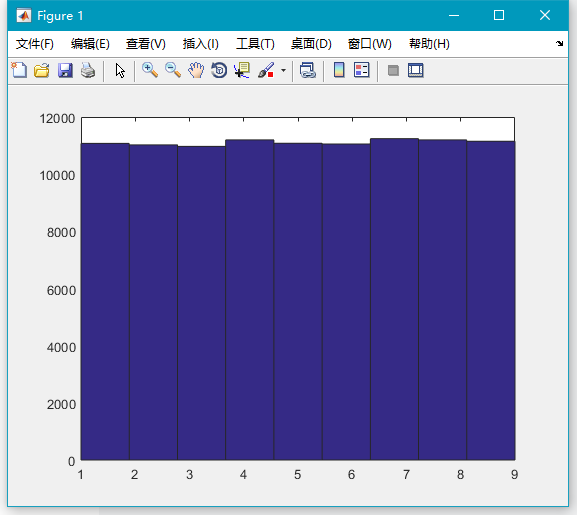### 15．binornd()

``binornd(n,p,[M,N,P,...])``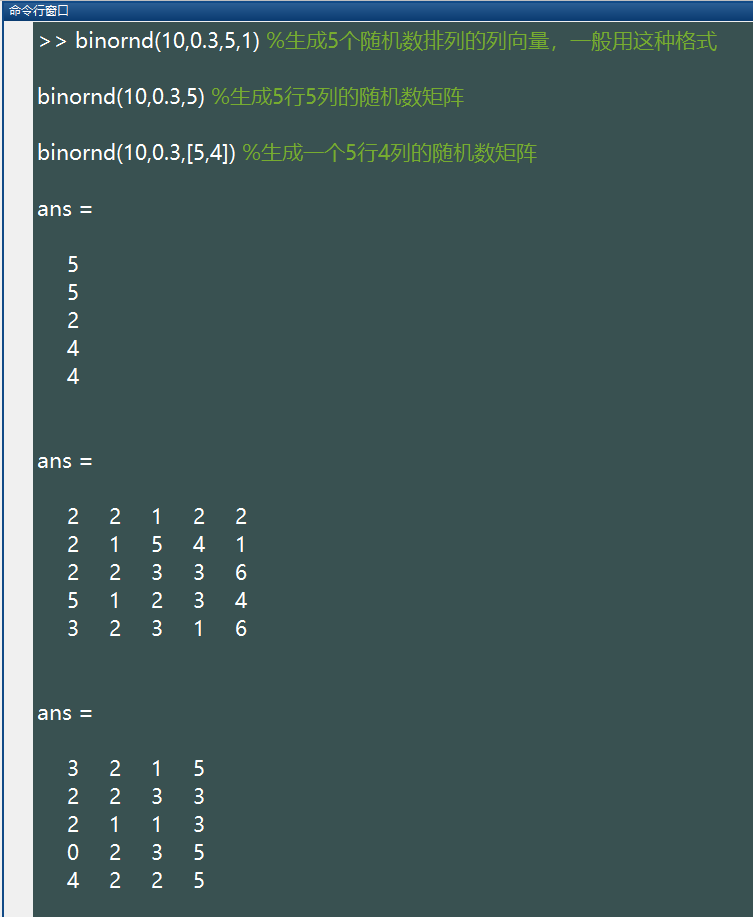%注：上述语句生成的随机数所服从的参数为 (10,0.3) 的二项分布

``````x=binornd(10,0.45,100000,1);

hist(x,11);``````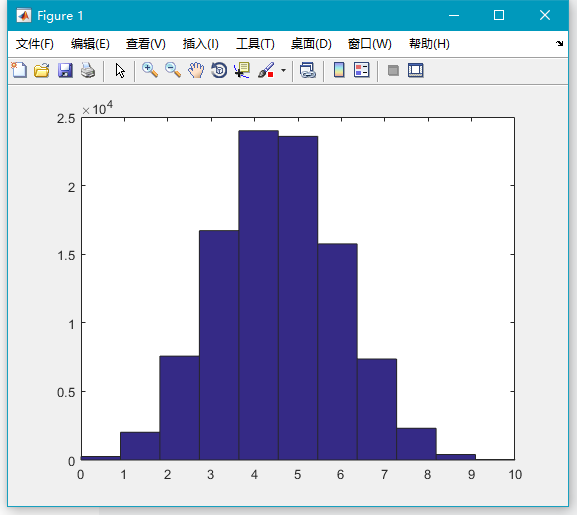### 16．geornd()

``geornd(p,[M,N,P,...])``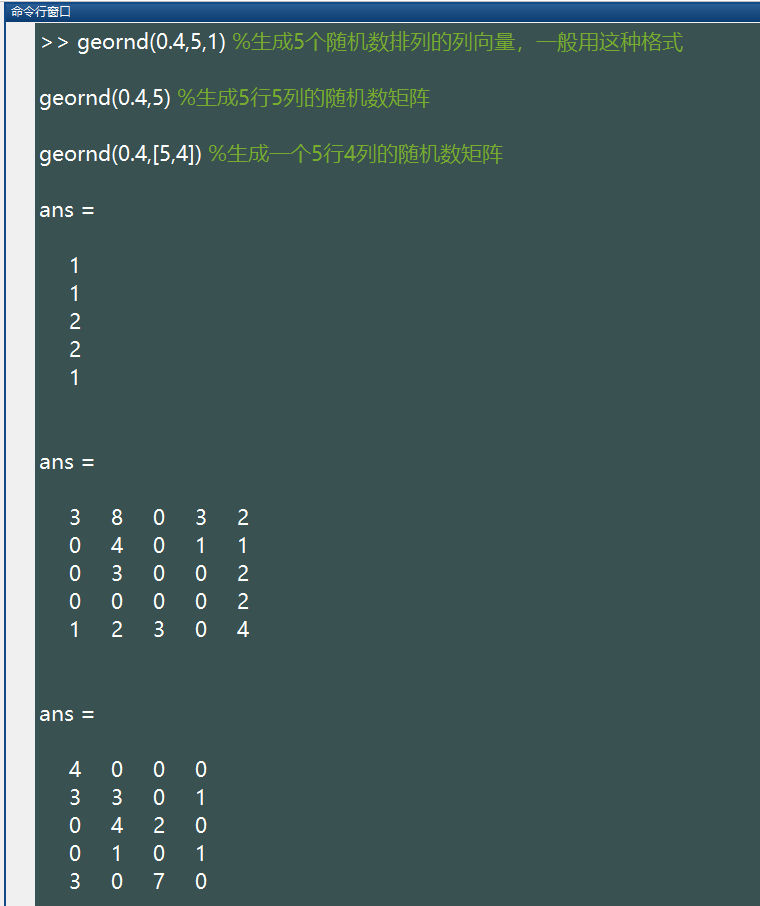%注：上述语句生成的随机数所服从的参数为(0.4)的二项分布

``````x=geornd(0.4,100000,1);

hist(x,50);``````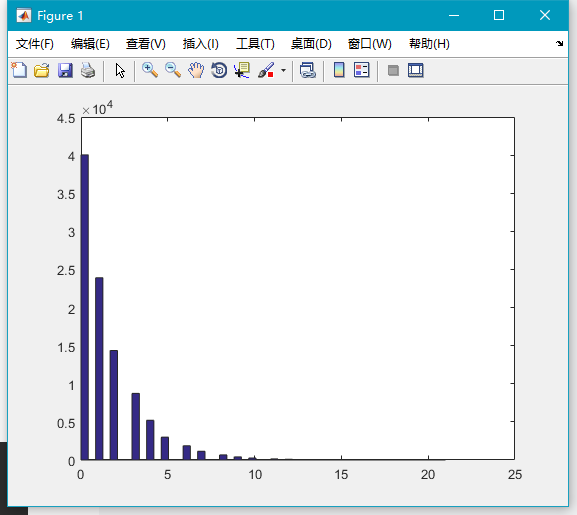### 17．poissrnd()

``geornd(p,[M,N,P,...])``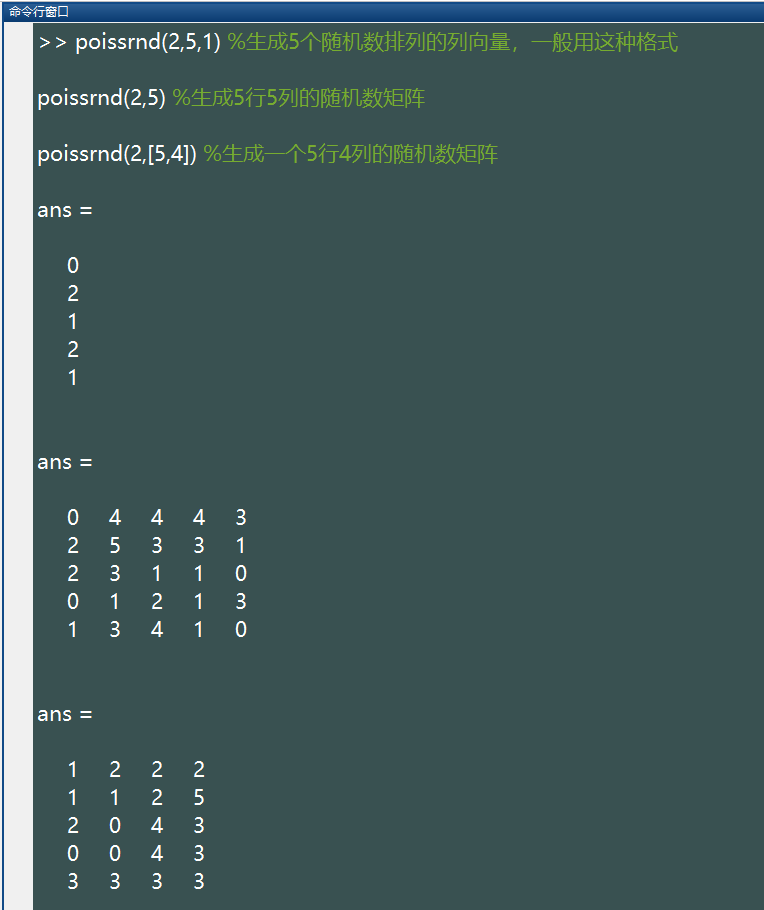%注：上述语句生成的随机数所服从的参数为(2)的泊松分布

``````x=poissrnd(2,100000,1);

hist(x,50);``````